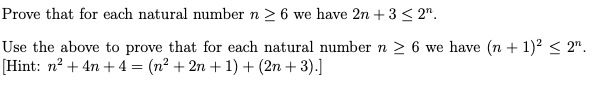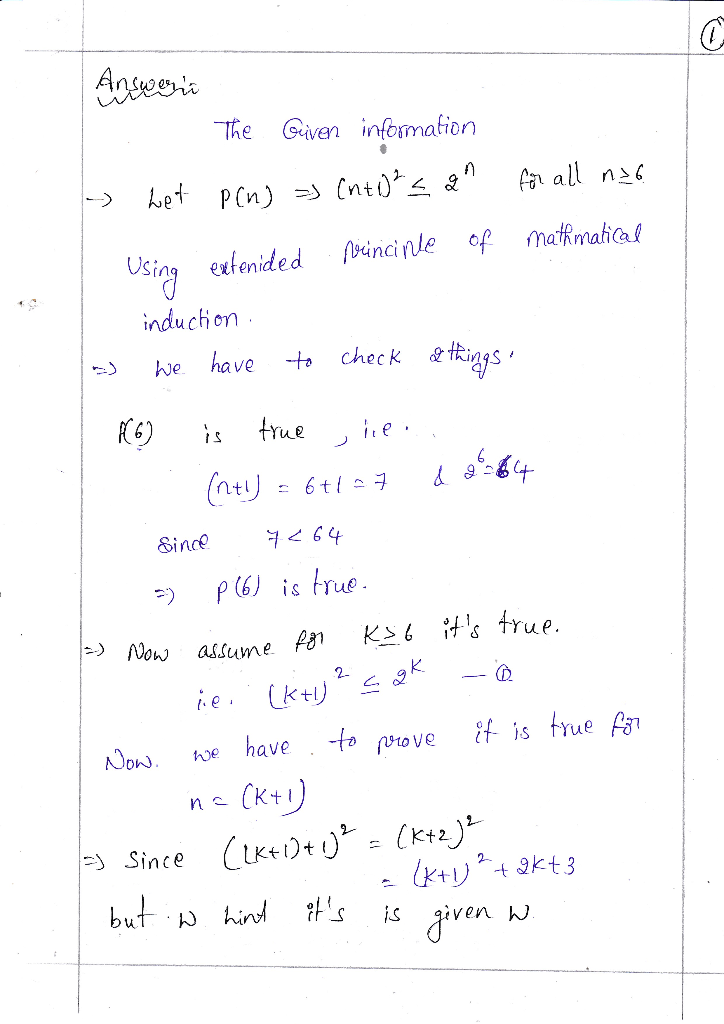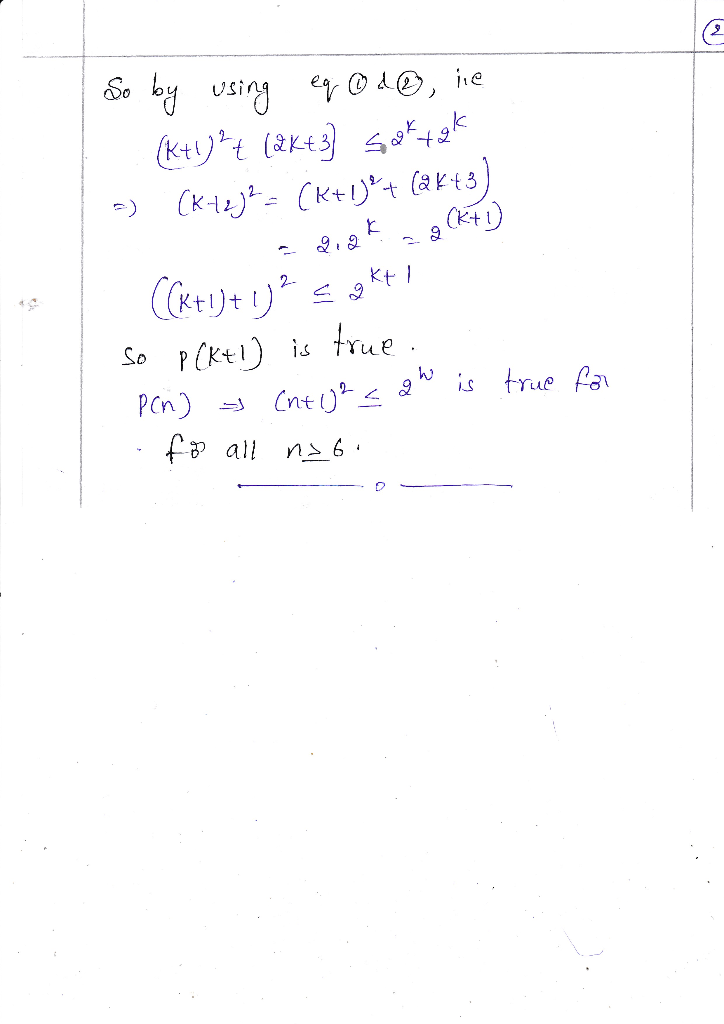Homework Help Question & Answers

# Prove that for each natural number n 26 we have 2n 3 3 2" Use the above to prove that for each na...Prove that for each natural number n 26 we have 2n 3 3 2" Use the above to prove that for each natural number n 2 6 we have (n +1)2 Hint: n24n +4-(n2 +2n +1) + (2n+3).] 2"

#### Homework Answers

ReportAnswer #1Add Homework Help Answer
##### Add Answer of: Prove that for each natural number n 26 we have 2n 3 3 2" Use the above to prove that for each na...
Your Answer: Your Name: What's your source?
Not the answer you're looking for? Ask your own homework help question. Our experts will answer your question WITHIN MINUTES for Free.
More Homework Help Questions Additional questions in this topic.

• #### 1. Above is the simple crosstab for Beta Blocker use vs. Low HDL. The natural log of the odds ratio [ln(OR)]= 2. Using the table from question 1, the standard error of ln(OR)= 3. Using the answer for...

Need Online Homework Help?

Get FREE EXPERT Answers
WITHIN MINUTES
Related Questions